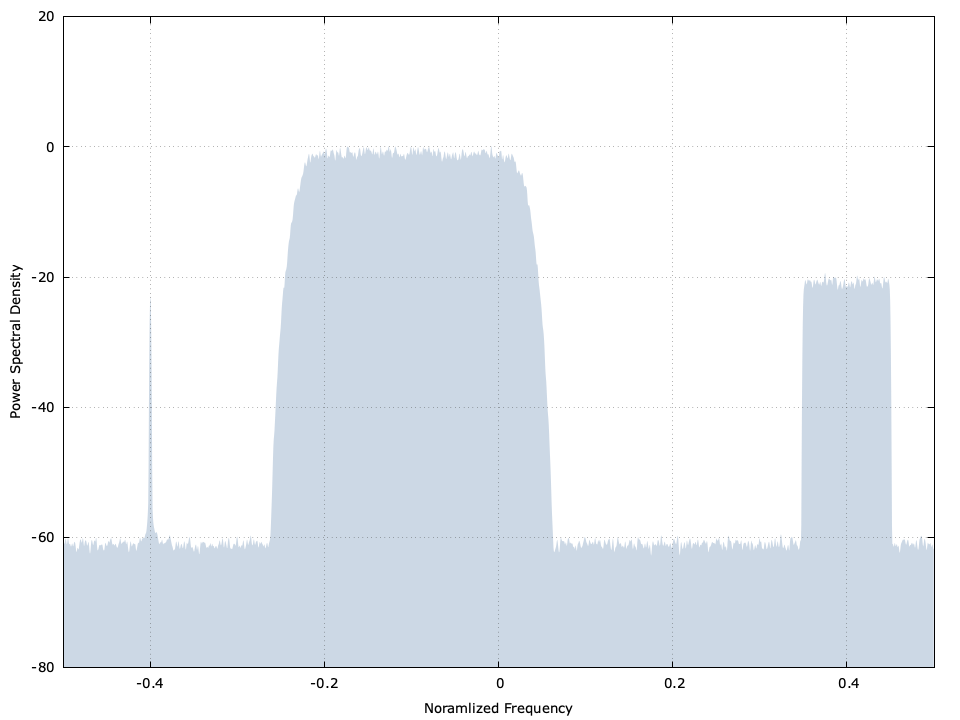# Multi-source signal generator (msource)

The msource family of objects are used to generate signals useful for testing communications systems. The interface allows for creating as many overlapping signals as you want, and provides a simple way to adjust their power level and center frequency independently with sample-level granularity.Figure [msource-example]. msourcecf example

## Interface

The msource object allows you to create the following signal types:

• id = msourcecf_add_tone(q) : add a discrete DC tone. To set the frequency of the tone, use the 'set frequency' method (see below for details)
• id = msourcecf_add_noise(q, bw) : add a noise source with a two-sided bandwidth bw .
• id = msourcecf_add_modem(q,ms,k,m,beta) : add a modulated data stream with the modulation scheme specified by ms , and interpolated with a square-root Nyquist filter ( LIQUID_FIRFILT_ARKAISER ) with k samples per symbol, a filter delay of m symbols, and an excess bandwidth factor beta . Internally the data stream is created with the symstream object.

You may add as many signal types as you like using the methods described above. Each invocation returns an integer identifier as a handle for manipulating properties of that particular signal type. For example, you may set the following properties for any signal type:

• msourcecf_set_gain(q,id,gain_dB) : set the signal gain in dB
• msourcecf_set_frequency(q,id,dphi) : set the signal's angular frequency relative to the sample rate. The value of dphi ranges from $$-\pi$$ to $$\pi$$ .

Additionally you may easily enable/disable each signal type using the following methods:

• msourcecf_enable(q,id) : enable signal
• msourcecf_disable(q,id) : disable signal

Generate samples to an output buffer of any size with msourcecf_write_samples(q,buf,buf_len) . When you're done with a signal, you can remove it from the object list with the msourcecf_remove(q,id) method.

## Example

The following listing creates the data in Figure [msource-example] at the top of this page. There are actually four signals: the tone at $$-0.4/F_s$$ , the modulated QPSK signal in the center, the narrow-band noise signal at $$+0.4/F_s$$ , and the wide-band noise signal spanning the full bandwidth. The signal is fed into a spectral periodogram object for estimating the signal's spectrum.


#include <stdio.h>
#include <stdlib.h>
#include <math.h>
#include <liquid/liquid.h>

int main()
{
// create stream generator
msourcecf gen = msourcecf_create();

// create spectral periodogram for estimating power spectral density
float alpha = 0.05f;
spgramcf periodogram = spgramcf_create_default(nfft);

unsigned int buf_len = 1024;
float complex buf[buf_len];

// add noise source (full-band)
int id =
msourcecf_set_gain(gen, id, -60.0f);

// add noise source (narrow-band)
id =
msourcecf_set_frequency(gen, id, 0.4*2*M_PI);
msourcecf_set_gain(gen, id, -20.0f);

id =
msourcecf_set_frequency(gen, id, -0.4*2*M_PI);
msourcecf_set_gain(gen, id, -40.0f);

// add modulated data (QPSK with 4 samples per symbol, a filter
// delay of 9 samples, and excess bandwidth of 30 %)
id =

// print source generator object
msourcecf_print(gen);

// generate 50 buffers worth of samples
unsigned int i;
for (i=0; i<50; i++) {
// write samples to buffer
msourcecf_write_samples(gen, buf, buf_len);

// push resulting sample through periodogram
spgramcf_accumulate_psd(periodogram, buf, alpha, buf_len);
}

// compute power spectral density output
float psd[nfft];
spgramcf_write_accumulation(periodogram, psd);

for (i=0; i<nfft; i++)
printf("  %12.8f %12.4e\n", (float)i/(float)nfft-0.5f, psd[i]);

// destroy objects
msourcecf_destroy(gen);
spgramcf_destroy(periodogram);

printf("done.\n");
return 0;
}